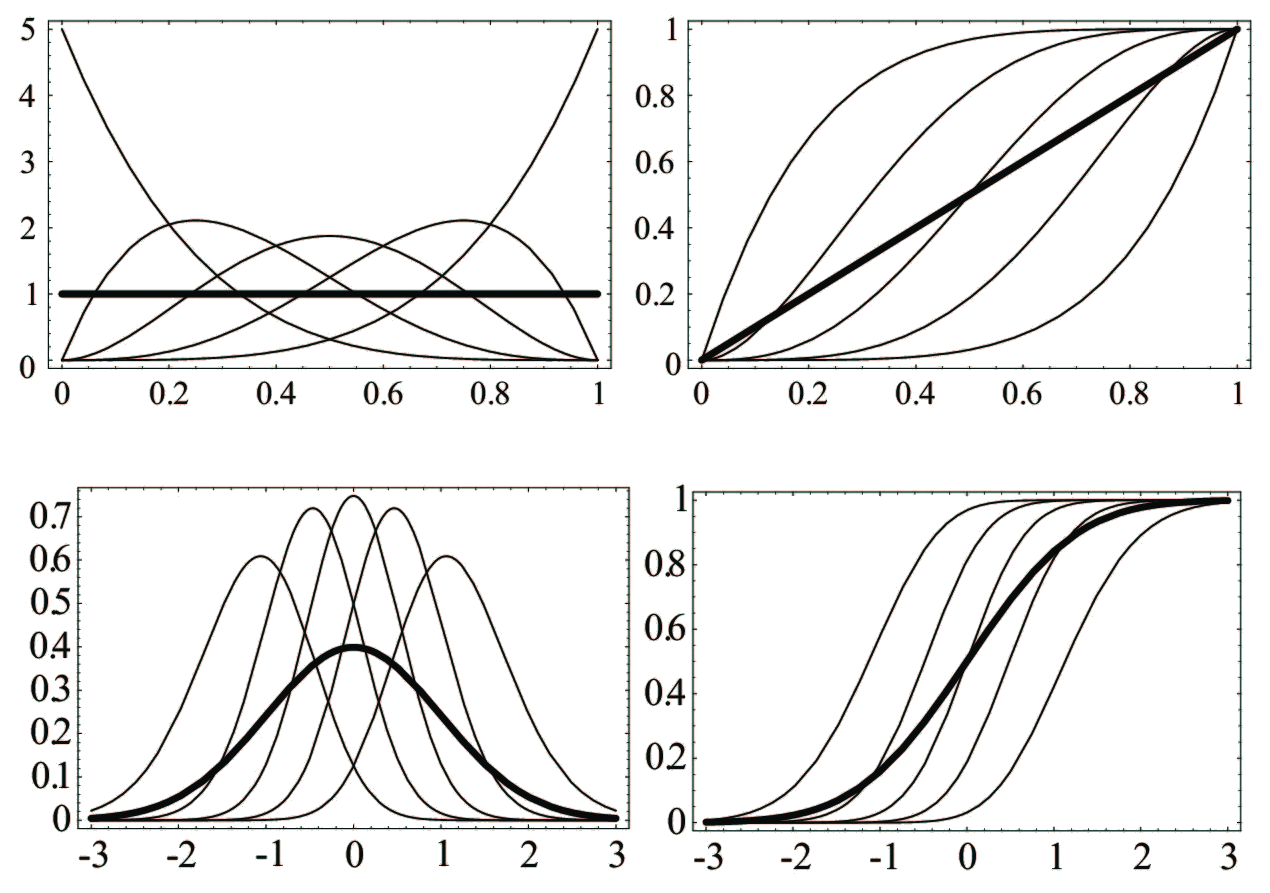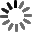El sitio web de la UCLM utiliza cookies propias y de terceros con fines técnicos y de análisis, pero no recaba ni cede datos de carácter personal de los usuarios. Sin embargo, puede haber enlaces a sitios web de terceros, con políticas de cookies distintas a la de la UCLM, que usted podrá aceptar o no cuando acceda a ellos.

Puede obtener más información en la Política de cookies. Aceptar# Extreme Values

Curso de fuentes OpenUCLM

In this course we start by introducing order statistics and extremes. In particular, the density functions of any order statistic or any subset of them are given in closed form. Next, we indicate that no matter the parent distribution is, the limit distribution of the maximum belongs to the Levy-Jenkinson family, which includes only the families of Weibull, Gumbel and Frechet. The corresponding limit distributions for the case of minimum are given too. The cases of other order statistics are also analyzed and discussed. The use of probability papers to find the most adequate limit distribution is explained and justified. Exceedances are recommended instead of extremes, because of its better efficiency and the generalized Pareto distribution is analyzed. The case of dependence is shown to lead to the same limits distributions if the dependence is not too strong, and to other limit distribution if it is strong enough. One illustrative example of extreme waves is studied in some detail.

Autores Fecha Enrique Castillo 17/09/2019 Ingles

Me GustaIn this first block we introduce the course and provide a motivation for it, based on engineering design.

A course on extreme values

Enrique Castillo

This video describes a course on extreme values
In this lesson extreme value distributions are motivated based on real examples from the engineering area. The differences between a standard statistical analysis and an extreme value analysis are pointed, emphasized and motivated.

We introduce the order statistics of a sample and the special order statistics minimum and maximum of the sample. We show how to obtain the joint distribution of any subset of order statistics, that includes the maximum, minimum and any particular order statistic or any subset of order statistics

Order Statistics

Enrique Castillo

We introduce the concept of order statistics and derive the joint density of any k order statistics. As particular cases, we derive the density of any order statistic, in particular the minimum and the maximum, the joint density of any two order statistics and the joint density of all order statistics. Some illustrative examples are given.
We study the limit distributions of extremes, minima and maxima, in the case of independence. It is shown that a unique family of distributions is possible for minima and another one for maxima. This family can be partitioned into the well known Weibull, Gumbel and Frechet fmilies of distributions. Some materials to work with extremes is given to the users.

We obtain the limit distributions for dependent random sequences and analyze when the limit coincides with the independence case. We also obtain the limit of not extreme order statistics and see the relation with non extreme cases

In this lesson we study the limit distributions of extremes for the case of dependent samples and provide conditions under which the limit distributions coincide with those for the case of independent samples. Some weak and more strong dependencies are described.
In this lesson we obtain the limit distributions of order statistics different from maxima and minima. We distinguish beteen central and low and high order statistics, showing that the limit behavior of these two order statistics is completely different. We also show that the sequences and the limit parameters for the extreme cases can appear in these cases.

It is explained how probability papers are constructed and how can they be used to solve important problems in engineering. Exceedances are introduced and recommended as better than minima and maxima to identify the extreme values.

Probability Papers

Enrique Castillo

We introduce probability papers and we show how to build probability papers for different families of distributions. In addition to explain the use of these papers to identify parents, we explain in detail how to use probability papers to identify domains of attractions, that is, which of the Weibull, Gumbel or Frechet families must be used for approximating extreme value distributions in practice.
This lesson explains how exceedances have advantages with respect to extreme values, because they provide much more information about the tails of the distributions. The generalized Pareto distribution is used to describe the exceedances over a certain threshold.
In addition, the concept of return period is introduced and the two different cases of exceedances and shortfalls treated.

In this block we present an interesting example in which the relevant variables to design a breakwater are modelled by a three-dimensional random variable including significant wave height, maximum wave height and period.

The example of a breakwater is used to illustrate the concepts introduced in this course. The example also aims to orient the readers to apply these concepts to engineering design of sea structures.

Conclusions

Enrique Castillo

Exercises

Enrique Castillo

Bibliography

Enrique Castillo

Here are all the slideshow from Extreme Values

Slideshows

Enrique Castillo